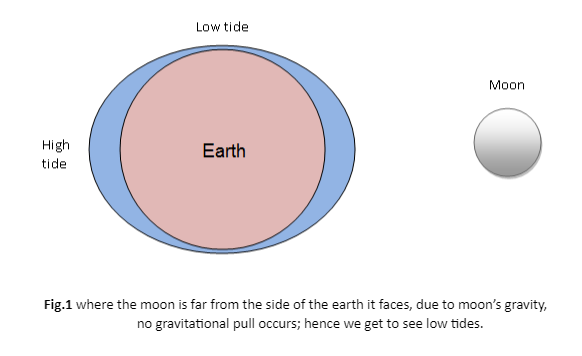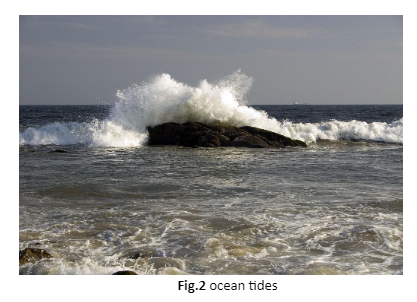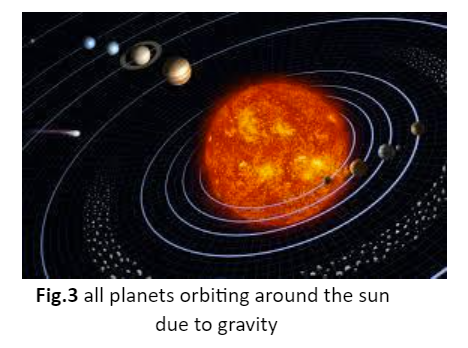• ### Browse All Lessons

##### Assign Lesson

Help Teaching subscribers can assign lessons to their students to review online!

 Tweet# Gravity

This lesson aligns with Next Generation Science Standards (NGSS) PS2.B

Introduction
When we pour water into a glass, it always stays at the bottom of the glass and never hovers over the top of the glass. Have you ever wondered, what makes the water stay at the bottom? Similarly, when a ball is thrown upward, it reaches a certain height and falls to the earth. It means that there is some kind of force present between the earth and the object that is responsible for dragging the object towards the center of the earth. The force observed is called gravity, which pulls the objects towards each other. In this article, we will learn about gravity, a universal law of gravitation, and the factors that affect gravity.

Gravity
Gravity is one of the fundamental forces in the universe which is defined as “a force of attraction that drags the objects towards the center of the earth”.

The gravitational pull between the earth and the moon generates tidal forces. When tidal forces act on the earth and water inside the ocean, as a result, the water bulges out from the side that is closest to the moon. Thus; high tides are observed in the ocean.Universal Law of Gravitation
In 1687, Newton was sitting under an apple tree. He noticed an apple falling from the tree. He figured out the situation and discovered the unknown force acting on an apple. What Newton concluded is known as a universal law of gravitation. Newton’s law of universal gravitation is stated as the gravitational force acts between all the objects in the universe. This means that every particle attracts another particle in the universe.

Gravity in the Universe
Gravity plays a major role in sustaining life in the universe. Gravity is the force that acts everywhere in the universe, not only on the earth. It keeps the moon orbiting around the earth and keeps all the planets orbiting around the sun as well.Factors Affect the Gravity
Gravity depends on the following factors.

Mass
Mass is a scalar quantity defined as “the measure of the amount of matter in a body”. The S.I. unit “kilogram” is used to measure the mass. Gravity between the objects increases with the greater mass. The more massive object is, the more gravitational pull it exerts. The mass of the earth is very great when compared to any person, while it is very small compared to the mass of the sun. This is the reason all the planets orbit around the sun.

Distance
Gravity is inversely proportional to the square of the separation between the objects. When two objects are farther apart from each other, gravity gets weaker. Similarly, when the distance decreases, the gravitational pull between the objects increases.

Weight
Weight is defined as the gravitational force exerted on the objects on the surface of the earth. The S.I. unit “newton” is used to measure the weight. Weight depends on the mass of the object and acceleration due to gravity. That’s why the objects that have greater mass, will have a greater weight. The weight of an object can be calculated by using the following formula;

Weight = mass×accleration due to gravity

As mentioned above, mass is measured in kilograms and the value of gravitational acceleration is $9.8 m//s^2$ on the surface of the earth. For instance, if we calculate the weight of a person having a mass of 20 kg, it will be $20 kg × 9.8 m//s^2 = 196 N$.

The weight of the objects varies with the strength of gravity. Our weight is six times less on the moon than it would be on earth due to a difference in the strength of gravity on the moon. For example, your weight on earth is 600 newtons. Then you will move to the moon and weigh yourself again. Your weight would be only 100 newtons.

Summary
• Gravity is a force that drags objects toward the center of the earth e.g. the water at the bottom of the glass does not hover to the top of the glass.
• The gravitational force depends on the mass and the distance between the objects.
• The massive an object is, the greater gravitational force is exerted on it. Similarly, when the distance between two objects increases, gravity decreases.
• The moon has one-sixth times the gravity of the earth. This is the reason why our weight is six times less on the moon as compared to the earth.

Related Worksheets: